# Chapter 10. Functions

JavaScript is a well-known functional language for programming. As the name hints, functions are an extremely important part of the language.

In this chapter, we will introduce you to functions and you will learn their definition, as well as what their base concepts are while working with them. You will learn why it's a good practice to use them, how to define and declare them. We will also introduce you to the parameters and return value of a function, as well as how to use that return value. In the end, we will peek at best practices when using functions.

## What is "function"?

Until this moment, we have found out that while writing a programming code for an exercise, separating the exercise into different parts, favors us a lot. Every part is responsible for a corresponding action and by doing so it's easier to solve the problem, and the readability and the debugging of the code are better.

A block of code designed to perform a particular task and which we have separated logically is called function. Functions – slices of code, that are named by us in a specific way. They can be called numerous times when we need them, and they will be run that many times as we want to.

One function can be called that many times, as we think is needed for solving a problem. That saves us from repeating the same code and reduces the opportunities of making a mistake while editing the repeated code.

### Simple functions

Simple functions are responsible for running a specific action, that will help us solve a problem. Those actions can be printing a string on the console, doing a conditional statement, doing a loop, etc.

Let's see an example of a simple function: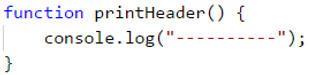This function has the task to print a header, that is a series of the symbol -. Because of this, the name of the function is printHeader. The round brackets ( and ) are always after the name, no matter how we have named the functions. Later we will take a look at how we have to name a function we are working with. For now, we will only say that the name of the function must define the action that is doing.

The body of the function consists of programming code, which is located between the curly brackets { and }. Between them, we place code, that will solve our problem, described by the name of the function.

### Why should we use functions?

Up to this moment, we have found out that, functions help us with separating long exercises into smaller parts, which leads to a simple solution of the corresponding problem. This makes our program, not just well structured, easy readable but also more understandable.

Using functions we escape repeating of programming code. Repeating code is a bad practice because it makes harder maintenance for the programmer to do which leads to errors. If one part of code exists in our program more than once and we have to fix it, we will have to change every occurrence of repeating code. The probability of us forgetting one of the repeated places is high, which will lead to incorrect behavior of our program. This is exactly why it's a good practice to define a fragment that will be used more than once as a separate function.

Functions offer us a good method to use code several times. With solving more and more exercises, we will come to the conclusion that using already defined functions saves us a lot of time and effort.

## Declaring functions

In Javascript language we can define functions everywhere, using the same way we define variables. Declaring represents the registration of a function inside a program and to be recognized inside it.

Javascript isn't strongly typed language. That's why when we declare a function it doesn't have a type(string, number, array, etc.), which other programming language methods have.

There are two ways, to declare a function in Javascript - function declaration and Function expression.

### Function declaration

With the next example, we will take a look at the required elements inside a function, using function declaration.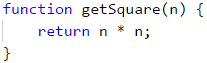• Keyword function. We start by using the keyword function, with which we define that there will be declared a function. We name it keyword because it is reserved in the Javascript language. We can't have a variable that is named function exactly because it is reserved.
• Name of the function. The name of the function is defined by us and we must never forget that it must define the task it is doing. In the example, the name getSquare tells us that the task of this function is to find the area of a square.
• List of parameters. We declare them between ( and ) brackets, which we type after the name of the function. Here we list a series of parameters, which the function will use. We can have only one parameter, more than one parameter or we can leave it empty. If there are no parameters we will only type the brackets (). In the current example, the parameter is only the n.
• Body of the function. It is declared between { and } brackets which we type after the closing bracket ). In our body of the function, we define all operations, that we want our function to do, using code. We also describe the algorithm by which the function will solve the given problem. We achieve the logic of the function. In the current example, we calculate the area of the square using n * n.

When declaring functions it is important to follow the sequence of the fundamental elements - first Keyword function then Name of function, List of parameters surrounded by round brackets (), Body of the function surrounded by curly brackets {}.

### Function expression

With the next example, we will take a look at the required elements in the declaration of an expression function. It is similar to function declaration which we have already viewed and it can be said that it's a set of declaring a variable and declaring a declaration function.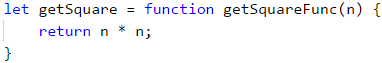• Keyword let. We will start with using the keyword let, with which we will declare a declaration of a variable.
• Name of the variable. The name of the variable is decided by us. In the example, the name is getSquare which tells us that the task of this function is to calculate the area of the square.
• Declaration of a function. Using the same structure that we have learned in function declaration - first Keyword function then Name of function, List of parameters surrounded by round brackets (), Body of the function surrounded by curly brackets {}. The difference, in this case, is that Name of a function is not necessary, but it is recommended to get used to writing the name. In the example, the program will work without problems even if we don't type getSquareFunc. If we don't type the name, the function will become anonymous.

When we declare a variable in the body of a function (using the keyword let or const). We call it a local variable for the function. The scope in which it exists and can be used is from the row it is defined to the end of the closing bracket } of the function. That scope is called variable scope.

### Function declaration or Function expression

The difference between Function declaration and Function expression is very simple. All functions declared by function declaration are loaded in the memory of a program before it is started. However, when we are using function expression the program will know and run the function only when it has come to it.

In theory, this means that we can call a function that is declared with function declaration even before it was declared in the previous rows. If we try to use function expression the program will throw an error that the function is not declared yet.

## Invoking a function

Invoking a function is a start of the execution of a code that is located inside the body of a function. We call it by typing the name of the function followed by () and ; to end the row. Here is an example: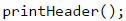This function can be called from different points in our program. One of the ways to be called is to be called from the global scope.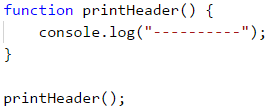A Function can be invoked from the body of another function which is not the global scope of our program.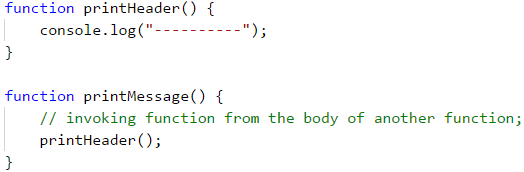A Function can be called from its own body. This is called recursion and you can find more about it on Wikipedia or google it.

### Example: empty cash receipt

Write a function, that prints empty cash receipt. The function must call another three functions: one to print the title, one for the main part, and the last for the bottom part.

Part of cash receipt Text
Upper part CASH RECEIPT
------------------------------
Middle part Charged to____________________
Bottom part ------------------------------
(c) SoftUni

#### Sample Input and Output

Input Output
None CASH RECEIPT
------------------------------
Charged to____________________
------------------------------
(c) SoftUni

#### Hints and Guidelines

Our first step is to create a function for creating a title. We should give it a short descriptive name. For example: printReceiptHeader. In the body we will write the following code: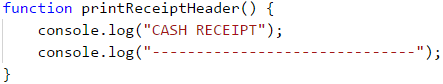In a like manner, we will create another two functions to print the middle part of the receipt (body) printReceiptBody and to print the bottom part of the receipt (footer printReceiptFooter.

After this we will set one more function* which will call the other three functions which we have written so far: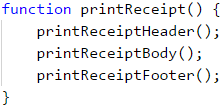In the end, we will invoke printReceipt from the global scope of our program: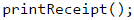#### Testing in the Judge System

The program with a total of four functions that call one another is ready and we can run and debug it. After that, we will send it for test in the Judge system: https://judge.softuni.org/Contests/Practice/Index/943#0.

## Functions with parameters

When we are dealing with a given task the function we are using needs additional information which the result depends on. Exactly this information are the parameters of the functions and the behavior of a program depends on.

### Using parameters inside a function

If the function requires input data, it is passed inside () brackets. The order of function parameters must match the order of the function arguments when declaring and invoking the function. Parameters can be zero, one, or more. When declaring the parameters we divide them with a comma ,.

Declaring function printNumbers(...) and the list of parameters which the programs need to work correctly will look like this: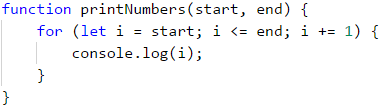After this, we invoke the function, and we give it the corresponding function arguments: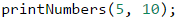When declaring function parameters we should check if every parameter has a name. It's also important when we invoke a function we should always pass values in the order that they were declared. In the previous example, the variable start will be passed to the first element (in our case the number 5). The variable end will have the next number which is 10.

It's important to point out that in the programming language JavaScript declaring a function with a given number of parameters doesn't force us to invoke a function with the same number of parameters. We can invoke the function by giving it more or fewer parameters than needed and it won't cause an error.

Let's look at this example:In this example, we call the function printNumbers(...) and we give 4 instead of the declared 2 parameters. All unnecessary parameters will be ignored. These are the numbers 15 and 20. They won't go to the function because the function doesn't have a declared parameter that will get them.

Let's look at another example: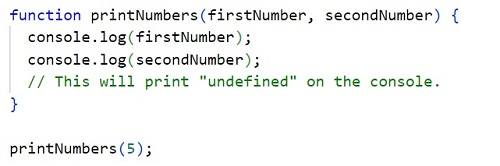In this example, we call the function printNumbers(...) but this time we give only 1 parameter instead of the declared 2 parameters. All parameters that are not set as values will automatically get undefined values. In our case the variable.

### Example: Symbol of integer

Create a function that checks if a number is a positive or negative number.

#### Sample Input and Output

Input Output
2 The number 2 is positive.
-5 The number -5 is negative.
0 The number 0 is zero.

#### Hints and Guidelines

Our first step is declaring a function and giving it describing name - printSign. This function will only have one parameter: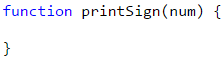Our next step is implementing logic by which our program will check if the number is positive or negative. From the example, we can see that there are 3 cases: if the number is bigger and smaller than zero or if it's zero. We will make three conditional statements in the body of our function.

Our next step is to call the function we have created: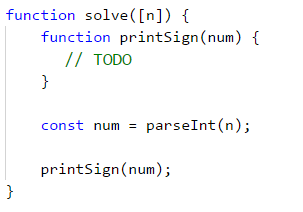### Optional parameters

JavaScript programming language supports optional parameters. They allow skipping of parameters when calling a function. We declare them by providing default values in the description of the parameter.

Our next example shows the use of optional parameters: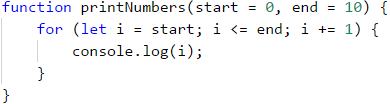printNumbers(...) can be invoked in different ways:When we are not setting value for the parameter, he will get the value that we have given him when declaring the function.

### Example: Print of triangle

Create a function that will create a triangle as shown in the example.

#### Sample Input and Output

Input OutPut Input OutPut
3 1
1 2
1 2 3
1 2
1
4 1
1 2
1 2 3
1 2 3 4
1 2 3
1 2
1

#### Hints and Guidelines

We choose a name according to the task it will do. For example, printLine and we implement it:From drawing on console exercises we remember that it's a good practice to divide the figure into different parts. We will divide the triangle into 3 parts - upper, middle, and bottom.

Our next step is to print the upper body of the triangle with a loop:After that, we will print the middle part:In the end, we will print the bottom part from the triangle but this time with a reverse loop.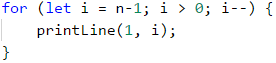### Example: draw a filled square.

Draw a square with side n, as shown in the example.

#### Sample Input and Output

Input Output Input Output
4 --------
-\/\/\/-
-\/\/\/-
--------
5 ----------
-\/\/\/\/-
-\/\/\/\/-
-\/\/\/\/-
----------

#### Hints and Guidelines

We create a function that prints the first and last row because they are the same. We shouldn't forget that we have to give him a corresponding name and set as a parameter the length of the side. We will use the built-in function repeat(...):Our next step is to create a function that will draw on the console the middle rows. Again we set a descriptive name such as printMiddleRow.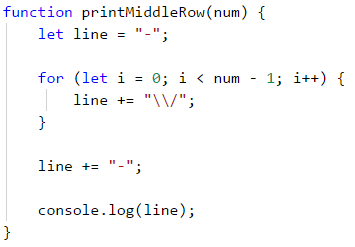In the end, we invoke the declared functions to draw the whole square: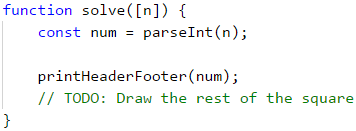## Return result from a function

Up to this point, we have viewed functions that do a specific task. For example printing a text, number or a figure on the console. There is another type of function that can return a result from their task. We can return a result of the multiplication of two numbers. We will look at this type of function in this part.

### Operator return

To get a result from a function we use the operator return. He must be used inside the body of a function. The return will tell the program to stop the execution of the function and return a corresponding value. This value is defined after the return word.

In the example underneath we have a function that gets the first and last name as parameters. Then it combines them and returns the full name.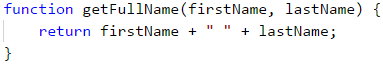There are cases in which return can be called from different places inside the function, but if only the conditions are met.

In the example below, we have a function that compares 2 numbers and returns the result which can be -1, 0, or 1 if the first argument is smaller, equal, or bigger than the second argument. The function uses the operator return on 3 different places. It returns different values depending on the logic of the code.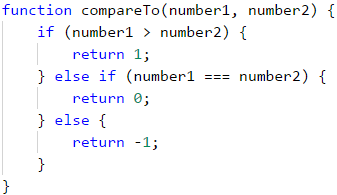#### Code after a return is unreachable

When the return operator is located inside a conditional statement such as if, after the statement in the same block, we must not have rows with code because Visual Studio Code will display a warning telling us that it had found an unreachable code.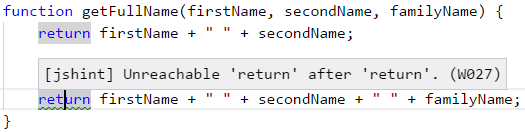The operator return can be also used without a specific value. In this case, the function will be terminated and the return value will be undefined.In programming, we can't have 2 times the operator return one after another because the first return won't allow us to use the second. Sometimes programmers joke with the phrase type return; return; and let's go home”, to explain that the logic of the program is wrongly typed.

### Using the return value of a function

After a function is executed and has returned a value, we can use the value in several ways.

The first is to assign the result to a variable: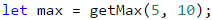The second is to be used inside an expression: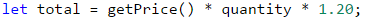The third is to pass the result from one function to another function: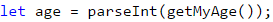### Example: Find the area of a triangle

Write a function that finds the area of a triangle by given side and altitude and then returns the area.

Input Output
3
4
6

#### Hints and Guidelines

We Create a function with a descriptive name.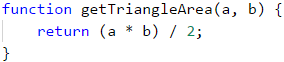Our next step is to call the new function and record the returned value inside another variable.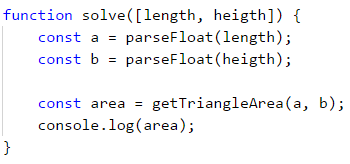### Example: Power of a number

Write a function that calculates and returns the result the power of a number.

#### Sample Input and Output

Input Output Input Output
2
8
256 3
4
81

#### Hints and Guidelines

Our first step is again to create a function that will get 2 parameters (base and exponent(power)). Then the function will return the result.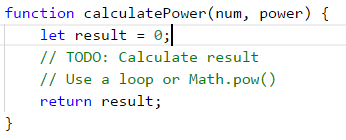After we have done our calculations, all we are left to do is calling the function.

### Functions, returning multiple values

In practice, there are some cases in which we need a function that returns more than one element as a result. In the Javascript language, there are 2 ways of doing that. They are with destruction and by returning an object.

#### Destruction

When we want a function to return more than one value, we use the keyword return and after that, we list all the values we want to return between [, ] brackets: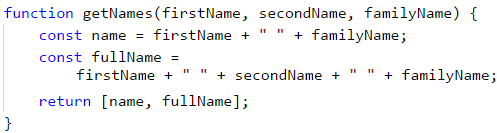After that to get the returned values using the square brackets we list a new set of variables that will get the returned values. We must follow the order we have returned them in the function.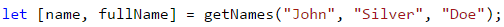In the upper example, the variable name will get the value "John Doe", which is the first of the returned function of getNames, and fullName will get "John Silver Doe*" which is second.

#### Objects

This way is very similar to the previous one. The only difference is that we don't just list all the values which we want to return. We give them names. Objects are extremely important and a big part of the Javascript language. For now, it's enough for us to know that they are declared with curly brackets { } and we type the name of the value (it's called a key). After the name, we type : followed by a value. We divide different key-value pairs with ,.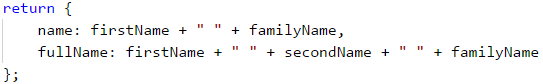In this example, we return an object which holds 2 values - name and fullName.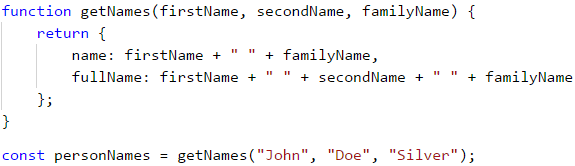Here the variable personNames will get all the returns values. By calling .name and .fullName we get the returned values: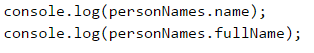## Variant of function

In many programming languages, one function can be declared as different variants with the same name but different parameters. This is known as method overloading. For good or bad Javascript, language doesn't support this.

When we declare two or more functions with the same names, our program will use the last declared. Declaring the second function with the same name removes the old function and overrides the new one in that place.

## Nested Functions

Let's take a look at this example: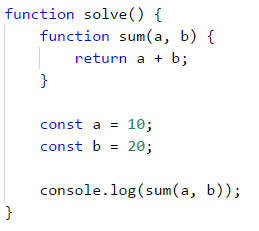### What is a local function?

We see that in the previous example, solve() function has another declared function sum(). This nested function is called a local function. Local functions can be declared in every other function.

### Why should we use local functions?

With time and practice we will find out that when we type code, we often need functions, which we might need only once or another function is becoming too long. We have already said that when one function has too many rows of code it is very hard to read, support, and understand. In those cases, we can declare another function which we will use even only once. This helps the code to be cleaner and reduces the chance of making a mistake in the programming code.

### Declaring local functions

Let's look again at the previous exampleIn this example sum() is local function because it's nested inside solve() function. This means that sum() can be called only inside solve() function because it's only declared inside it.

Local functions have access to variables, which are declared in the same or upper level from them. Our next example shows how this happens.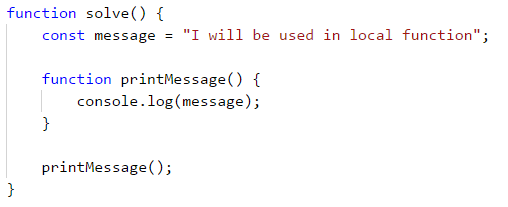This feature of nested functions makes them convenient helpers when solving a task. They save time and code by not having to pass values to parameters and variables.

## Naming functions. Best practices for working with functions.

In this part, we will take a look at some of the best practices for writing functions that are connected to an arrangement of a code and its structure.

### Naming functions

When we name a function it's recommended to use logical names. This is good because every function must correspond to a specific part of our problem. We must take into consideration the task which the function will do. This is why it's a good practice for the name to describes the purpose of the function.

It is required for the name of the function to start with a small letter and to be a verb or a combination of a verb and a noun. Formatting the names must be done following Lower Camel Case convention - every word except the first to start with an upper letter. Round brackets ( and ) are always after the name of the function.

Every function must do an independent task and the name must describe its role.

Here are some examples of correctly named functions:

• findStudent
• loadReport
• sine

Some examples of badly named functions:

• method1
• doSomething
• handleStuff
• sampleMethod
• dirtyHack
• FindStudent
• LoadReport

If we can't come up with a good name it's probably because our function does more than one job or it doesn't have a clear task. We must think of a way to divide the function into different functions.

### Naming parameters of functions

When naming parameters of functions, the same rules of naming functions apply to them. The only difference is that the name of the parameters should be nouns or a set of a noun and an adjective. It's a good practice that the name of the parameter should indicate what type of unit is used when working with it.

Here are some examples of correctly named parameters of functions:

• firstName
• report
• speedKmH
• usersList
• fontSizeInPixels
• font

Some examples of incorrectly named parameters:

• p
• p1
• p2
• populate
• LastName
• last_name

### Good practices for working with functions

We must keep in mind that a function must do only one specific task. If this can't be achieved, then we must come up with a way to divide the functionality into different parts. As we have already said the name must be clear and descriptive. Another good practice is to avoid functions that are longer than our screen. If this happens it's recommended to split the function into smaller ones as shown in the example below.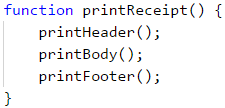### Structure and formatting of the code

When writing functions we must keep in mind to follow a correct indication (move more inward blocks of the code).

Here are some examples of correctly formatted Javascript code: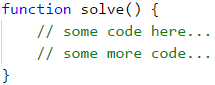Some examples of incorrectly formatted Javascript code: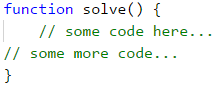When the headline row of the function is too long, it's recommended to be split into several rows, and every row after the first should be moved 2 tabulations to right (for clear readability).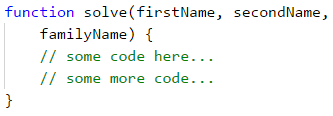Another good practice is to leave an empty row between functions, after loops, and conditional statements. You should always try to avoid writing long rows and complicated expressions. Over time you will find out that improves the readability and saves time.

We recommend you to always use curly brackets for the body of conditional statements and loops. The brackets don't just improve the readability but also reduce the possibility of making a mistake.

## What have we learned from this chapter?

In this chapter we have learned base concepts about working with functions:

• Learned that the goal of a function is to split programs with many rows to code with smaller and shorter parts.
• Comprehended the idea of the structure of functions and how to declare and invoke them by their name.
• Looked into different examples of functions with parameters and how to use them in our program.
• Learned what is signature and return value of the function as well as what is the role of the return operator.
• Studied good practices for working with functions, how to name them and their parameters and how to format our code and others.

## Exercises

To improve what we have learned we will solve a few exercises. In them, it is required to write functions with specific functionality and then call it with values as shown in the example.

### Problem: "Hello, Name!"

Write a function that takes a name as a parameter and prints on the console "Hello, {name}!".

#### Sample Input and Output

Input Output
Peter Hello, Peter!

#### Hints and Guidelines

Define a function printName(name) and implement it. Write a function solve(...) which receives as input name of a person and calls printName function.

### Problem: Min Method

Define a function GetMin(int a, int b) and implement it, after which invoke it from the function solve(...) as shown below. To find the minimum of three numbers, first, find the minimum of the first two and then the minimum of the result and the third number:

#### Sample Input and Output

Input Output Input Output
1
2
3
1 -100
-101
-102
-102

#### Hints and Guidelines

Define function getMin(int a, int b) and implement it, after that call it from solve(...) as shown in the example below. To find the minimum of the tree numbers find first the minimum of the first and second value. Then find the minimum of the result of the two and the third number:

function solve([num1, num2, num3]) {
let min = getMin(getMin(num1, num2), num3);
}


### Problem: String Repeater

Write a function repeatString(str, count) which gets as parameters string variable str and number n then returns the string repeated n times. After that print the result on the console.

#### Sample Input and Output

Input Output Input Output
str
2
strstr roki
6
rokirokirokirokirokiroki

#### Hints and Guidelines

Write function and add the input string to the result in the for loop: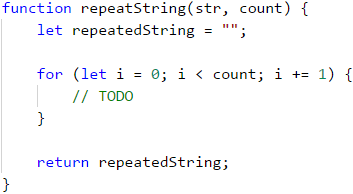### Problem: Nth Digit

Write function findNthDigit(number, index) which gets number and index then print the Nth digit of the number (starting to count from left to right from 1). After that print the result to the console.

#### Sample Input and Output

Input Output Input Output Input Output
83746
2
4 93847837
6
8 2435
4
2

#### Hints and Guidelines

To perform the algorithm we will use a while loop. While the number isn't 0 we will check if the index matches our input value. If it matches we will return the number of the index (number % 10). If it doesn't match anything we will remove the last digit of the number (number = number / 10). We must follow which digit we check in the conditional statement (starting from left to right from 1). When we find the digit we will return the index.

### Problem: Integer to Base

Write a function integerToBase(number, toBase), which takes as parameters an integer and a base of a numeral system and returns the integer converted to the given numeral system. After this, the result should be printed on the console. The input number will always be in the decimal numeral system, and the base parameter will be between 2 and 10.

## Sample Input and Output

Input Output Input Output Input Output
3
2
11 4
4
10 9
7
12

## Hints and Guidelines

To solve the problem, we will declare a string, in which we will keep the result. After this, we need to do the following calculations to convert the number.

• Calculate the remainder of the number, divided by the base.
• Insert the remainder at the beginning of the string.
• Divide the number to the base.
• Repeat the algorithm until the input integer equals 0.

Write the missing logic in the function below:

function integerToBase(number, toBase) {
string result = "";
while (number !== 0) {
// implement the missing conversion logic
}
return result;
}


#### Testing in the Judge System

Write a function solve(...), which takes an integer n and n input messages and prints n output messages, based on the input. For each message read a few lines. Each message starts with messageType: “success”, “warning” or “error”:

• When messageType is “success” read operation + message (each from a new line).
• When messageType is a “warning” read-only message (from a new line).
• When messageType is “error” read operation + message + errorCode (each from a new line).

Print on the console each read message formatted depending on its messageType. After the headline of the message print as much =, as the length of the said headline and print an empty line after each message (to understand in detail look at the examples).

The problem should be solved by defining four functions: showSuccessMessage(), showWarningMessage(), showErrorMessage() and processMessage(), so that only the last function is invoked by the Main() function: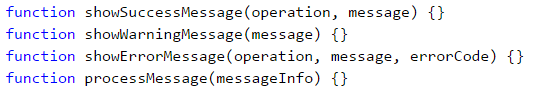#### Sample Input and Output

Input Output
4
error
credit card purchase
500
warning
Email not confirmed
success
user registration
User registered successfully
warning
Customer has not email assigned
Error: Failed to execute credit card purchase.
==============================================
Reason: Invalid customer address.
Error code: 500.

Warning: Email not confirmed.
=============================

Successfully executed user registration.
========================================
User registered successfully.

Warning: Customer has no email assigned.
=========================================

#### Hints and Guidelines

Define and implement the four shown functions. After that call processMessage(...) from the main solve(...) function.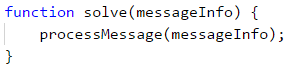In processMessage() read the type of message from the console and according to the read, type read the rest of the data (one, two, or three more lines). After that invoke the function for printing the given type of message.

### Problem: Numbers to Words

Write a function letterize(number), which reads an integer and prints it in words in English according to the conditions below:

• Print in words the hundreds, the tens, and the ones (and the eventual minus) according to the rules of the English language.
• If the number is larger than 999, you must print "too large".
• If the number is smaller than -999, you must print "too small".
• If the number is negative, you must print "minus" before it.
• If the number is not built up of three digits, you shouldn't print it.

#### Sample Input and Output

Input Output Input Output
3
999
-420
1020
nine-hundred and ninety nine
minus four-hundred and twenty
too large
2
15
350
fifteen
three-hundred and fifty
Input Output Input Output
4
311
418
509
-9945
three-hundred and eleven
four-hundred and eighteen
five-hundred and nine
too small
3
500
123
9
five-hundred
one-hundred and twenty-three
nine

#### Hints and Guidelines

We can first print the hundreds as a text – (the number / 100) % 10, after that the tens – (the number / 10) % 10 and at the end the ones – (the number % 10).

The first special case is when the number is exactly rounded to 100 (e.g. 100, 200, 300 etc...). In this case we print "one-hundred", "two-hundred", "three-hundred" etc.

The second special case is when the number formed by the last two digits of the input number is less than 10 (e.g. 101, 305, 609, etc...). In this case, we print "one-hundred and one", "three-hundred and five", "six-hundred and nine" etc.

The third special case is when the number formed by the last two digits of the input number is larger than 10 and smaller than 20 (e.g. 111, 814, 919, etc.). In this case, we print "one-hundred and eleven", "eight-hundred and fourteen", "nine-hundred and nineteen" etc.

### Problem: String Encryption

Write a function encrypt(char letter), which encrypts a given letter in the following way:

• It takes the first and the last digit from the ASCII code of the letter and concatenates them into a string, which will represent the result.
• At the beginning of the string, which represents the result, we will insert the symbol which matches the following condition:
• ASCII code of the letter + the last digit of the ASCII code of the letter.
• After that at the end of the string, which represents the result, you concatenate the character which matches the following condition:
• ASCII code of the letter - the first digit of the ASCII code of the letter.
• The function should return the encrypted string.

Example:

• j → p16i
• ASCII code of j is 106 → First digit – 1, last digit – 6.
• We concatenate the first and the last digit → 16.
• At the beginning of the string, which represents the result, concatenate the symbol, which you get from the sum of the ASCII code + the last digit → 106 + 6 → 112 → p.
• At the end of the string, which represents the result, concatenate the symbol, which you get from subtracting the ASCII code – the first digit → 106 - 1 → 105 → i.

Using the function shown above, write a function solve(...) which takes a sequence of characters, encrypts them, and prints the result on one line. The input data will always be valid. The Main function must read the data given by the user – an integer n, followed by a character for each of the following n lines. Encrypt the symbols and add them to the encrypted string. In the end, as a result, you must print an encrypted string as in the following example.

Example:

• S, o, f, t, U, n, i → V83Kp11nh12ez16sZ85Mn10mn15h

#### Sample Input and Output

Input Output
7
S
o
f
t
U
n
i
V83Kp11nh12ez16sZ85Mn10mn15h
Input Output
7
B
i
r
a
H
a
x
H66<n15hv14qh97XJ72Ah97xx10w

#### Hints and Guidelines

Our variable result in which we will save the value of the result we will give the initial value "". We must recur a loop n times so that in each iteration we will add the encrypted symbol to the result string.

To find the first and the last digit of the ASCII code, we will use the same algorithm that we used to solve "Integer to Base".